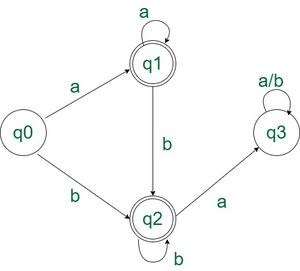GeeksforGeeks App
Open AppBrowser
Continue

# Program to construct a DFA which accepts the language having all ‘a’ before all ‘b’

Given a string S, the task is to design a Deterministic Finite Automata (DFA) for accepting the language L = {aNbM | N ≥ 0, M ≥ 0, N+M ≥ 1}. , i.e., a regular language L such that all ‘a’ occur before the first occurrence of ‘b’ {a, ab, aab, bb…, }. If the given string follows the given language L, then print “Accepted”. Otherwise, print “Not Accepted”.

Examples

Input: S = “aabbb”
Output: Accepted
Explanation: All the ‘a’ come before ‘b’ s.

Input: S = “ba”
Output: Not Accepted
Explanation: ‘b’ comes before ‘a’.

Input: S = “aaa”
Output: Accepted
Explanation: Note that ‘b’ does not need to occur in S

Input: S = “b”
Output: Accepted
Explanation: Note that ‘a’ does not need to occur in S

Approach: The problem can be accepted only when the following cases are met:

• All the characters can be ‘a’.
• All the characters can be ‘b’.
• All the ‘b’ come occur after all the ‘a’.
• There is at least one character in the string.

This can be better visualized with the help of the state transition diagram of the DFA

State Transition Diagram:State Transition Diagram of the above DFA

Below is the implementation of the above approach:

## C++

 `// C++ Program to implement``// the above approach``#include ``using` `namespace` `std; ` `// Function for state zero Q0``int` `startStateQ0(``char` `s) {``    ` `    ``int` `state;``    ``if` `(s == ``'a'``)``        ``state = 1;``    ``else` `if` `(s == ``'b'``)``        ``state = 2;``    ``else``        ``state = -1;` `    ``return` `state;``}``        ` `// Function for first state Q1``int` `firstStateQ1(``char` `s) {``    ` `    ``int` `state;``    ``if` `(s == ``'a'``)``        ``state = 1;``    ``else` `if` `(s == ``'b'``)``        ``state = 2;``    ``else``        ``state = -1;``        ` `    ``return` `state;``}``        ` `// Function for second state Q2``int` `secondStateQ2(``char` `s) {``    ` `    ``int` `state;``    ``if` `(s == ``'b'``)``        ``state = 2;``    ``else` `if` `(s == ``'a'``)``        ``state = 3;``    ``else``        ``state = -1;``        ` `    ``return` `state;``}``        ` `// Function for third state Q3``int` `thirdStateQ3(``char` `s) {``    ` `    ``int` `state = 3;``    ``return` `state;``}``        ` `// Function to check``// if the string is accepted or not``int` `isAcceptedString(string String) {``    ` `    ``int` `l = String.length();` `    ``// dfa tells the number associated``    ``// with the present dfa = state``    ``int` `state = 0;``    ``for` `(``int` `i = 0; i < l; i++) {``        ``if` `(state == 0)``            ``state = startStateQ0(String[i]);``        ``else` `if` `(state == 1)``            ``state = firstStateQ1(String[i]);``        ``else` `if` `(state == 2)``            ``state = secondStateQ2(String[i]);``        ``else` `if` `(state == 3)``            ``state = thirdStateQ3(String[i]);``        ``else``            ``return` `0;``    ``}``    ``if` `(state == 1 || state == 2)``        ``return` `1;``    ``else``        ``return` `0;``}` `int` `main() {``    ` `    ``string String = ``"ba"``;``    ``if` `(isAcceptedString(String))``        ``cout << ``"ACCEPTED"``;``    ``else``        ``cout << ``"NOT ACCEPTED"``;``}    ` `// This code is contributed by Samim Hossain Mondal.`

## Java

 `// Java Program to implement``// the above approach``import` `java.util.*;``public` `class` `GFG {` `    ``// Function for state zero Q0``    ``static` `int` `startStateQ0(``char` `s)``    ``{` `        ``int` `state;``        ``if` `(s == ``'a'``)``            ``state = ``1``;``        ``else` `if` `(s == ``'b'``)``            ``state = ``2``;``        ``else``            ``state = -``1``;` `        ``return` `state;``    ``}` `    ``// Function for first state Q1``    ``static` `int` `firstStateQ1(``char` `s)``    ``{` `        ``int` `state;``        ``if` `(s == ``'a'``)``            ``state = ``1``;``        ``else` `if` `(s == ``'b'``)``            ``state = ``2``;``        ``else``            ``state = -``1``;` `        ``return` `state;``    ``}` `    ``// Function for second state Q2``    ``static` `int` `secondStateQ2(``char` `s)``    ``{` `        ``int` `state;``        ``if` `(s == ``'b'``)``            ``state = ``2``;``        ``else` `if` `(s == ``'a'``)``            ``state = ``3``;``        ``else``            ``state = -``1``;` `        ``return` `state;``    ``}` `    ``// Function for third state Q3``    ``static` `int` `thirdStateQ3(``char` `s)``    ``{` `        ``int` `state = ``3``;``        ``return` `state;``    ``}` `    ``// Function to check``    ``// if the string is accepted or not``    ``static` `int` `isAcceptedString(String Str)``    ``{` `        ``int` `l = Str.length();` `        ``// dfa tells the number associated``        ``// with the present dfa = state``        ``int` `state = ``0``;``        ``for` `(``int` `i = ``0``; i < l; i++) {``            ``if` `(state == ``0``)``                ``state = startStateQ0(Str.charAt(i));``            ``else` `if` `(state == ``1``)``                ``state = firstStateQ1(Str.charAt(i));``            ``else` `if` `(state == ``2``)``                ``state = secondStateQ2(Str.charAt(i));``            ``else` `if` `(state == ``3``)``                ``state = thirdStateQ3(Str.charAt(i));``            ``else``                ``return` `0``;``        ``}``        ``if` `(state == ``1` `|| state == ``2``)``            ``return` `1``;``        ``else``            ``return` `0``;``    ``}` `    ``public` `static` `void` `main(String args[])``    ``{` `        ``String Str = ``"ba"``;``        ``if` `(isAcceptedString(Str) != ``0``)``            ``System.out.println(``"ACCEPTED"``);``        ``else``            ``System.out.println(``"NOT ACCEPTED"``);``    ``}``}` `// This code is contributed by Samim Hossain Mondal.`

## Python3

 `# Function for state zero Q0``def` `startStateQ0(s):``    ``if` `(s ``=``=` `'a'``):``        ``state ``=` `1``    ``elif` `(s ``=``=` `'b'``):``        ``state ``=` `2``    ``else``:``        ``state ``=` `-``1``    ``return` `state` `# Function for first state Q1``def` `firstStateQ1(s):``    ``if` `(s ``=``=` `'a'``):``        ``state ``=` `1``    ``elif` `(s ``=``=` `'b'``):``        ``state ``=` `2``    ``else``:``        ``state ``=` `-``1``    ``return` `state` `# Function for second state Q2``def` `secondStateQ2(s):``    ``if` `(s ``=``=` `'b'``):``        ``state ``=` `2``    ``elif` `(s ``=``=` `'a'``):``        ``state ``=` `3``    ``else``:``        ``state ``=` `-``1``    ``return` `state` `# Function for third state Q3``def` `thirdStateQ3(s):``    ``state ``=` `3``    ``return` `state` `#Function to check``#if the string is accepted or not``def` `isAcceptedString(String):``    ``l ``=` `len``(String)` `    ``# dfa tells the number associated``    ``# with the present dfa = state``    ``state ``=` `0``    ``for` `i ``in` `range``(l):``        ``if` `(state ``=``=` `0``):``            ``state ``=` `startStateQ0(String[i])``        ``elif` `(state ``=``=` `1``):``            ``state ``=` `firstStateQ1(String[i])``        ``elif` `(state ``=``=` `2``):``            ``state ``=` `secondStateQ2(String[i])``        ``elif` `(state ``=``=` `3``):``            ``state ``=` `thirdStateQ3(String[i])``        ``else``:``            ``return` `0``    ``if``(state ``=``=` `1` `or` `state ``=``=` `2``):``        ``return` `1``    ``else``:``        ``return` `0` `# Driver code``if` `__name__ ``=``=` `"__main__"``:` `    ``String ``=` `"ba"``    ``if` `(isAcceptedString(String)):``        ``print``(``"ACCEPTED"``)``    ``else``:``        ``print``(``"NOT ACCEPTED"``)`

## C#

 `// C# Program to implement``// the above approach``using` `System;``class` `GFG {` `    ``// Function for state zero Q0``    ``static` `int` `startStateQ0(``char` `s)``    ``{` `        ``int` `state;``        ``if` `(s == ``'a'``)``            ``state = 1;``        ``else` `if` `(s == ``'b'``)``            ``state = 2;``        ``else``            ``state = -1;` `        ``return` `state;``    ``}` `    ``// Function for first state Q1``    ``static` `int` `firstStateQ1(``char` `s)``    ``{` `        ``int` `state;``        ``if` `(s == ``'a'``)``            ``state = 1;``        ``else` `if` `(s == ``'b'``)``            ``state = 2;``        ``else``            ``state = -1;` `        ``return` `state;``    ``}` `    ``// Function for second state Q2``    ``static` `int` `secondStateQ2(``char` `s)``    ``{` `        ``int` `state;``        ``if` `(s == ``'b'``)``            ``state = 2;``        ``else` `if` `(s == ``'a'``)``            ``state = 3;``        ``else``            ``state = -1;` `        ``return` `state;``    ``}` `    ``// Function for third state Q3``    ``static` `int` `thirdStateQ3(``char` `s)``    ``{` `        ``int` `state = 3;``        ``return` `state;``    ``}` `    ``// Function to check``    ``// if the string is accepted or not``    ``static` `int` `isAcceptedString(``string` `Str)``    ``{` `        ``int` `l = Str.Length;` `        ``// dfa tells the number associated``        ``// with the present dfa = state``        ``int` `state = 0;``        ``for` `(``int` `i = 0; i < l; i++) {``            ``if` `(state == 0)``                ``state = startStateQ0(Str[i]);``            ``else` `if` `(state == 1)``                ``state = firstStateQ1(Str[i]);``            ``else` `if` `(state == 2)``                ``state = secondStateQ2(Str[i]);``            ``else` `if` `(state == 3)``                ``state = thirdStateQ3(Str[i]);``            ``else``                ``return` `0;``        ``}``        ``if` `(state == 1 || state == 2)``            ``return` `1;``        ``else``            ``return` `0;``    ``}` `    ``public` `static` `void` `Main()``    ``{` `        ``string` `Str = ``"ba"``;``        ``if` `(isAcceptedString(Str) != 0)``            ``Console.Write(``"ACCEPTED"``);``        ``else``            ``Console.Write(``"NOT ACCEPTED"``);``    ``}``}` `// This code is contributed by ukasp.`

## Javascript

 ``

Output

`NOT ACCEPTED`

Time Complexity: O(N) where N is the length of the string
Auxiliary Space: O(1)

My Personal Notes arrow_drop_up